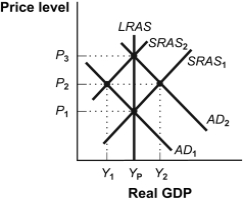# [Solved] Use the Following to Answer Questions

Question 227
Multiple Choice

## Use the following to answer questions:-(Figure: An Increase in Aggregate Demand)Refer to Figure: An Increase in Aggregate Demand.At the Y2 level of real GDP:

A) an inflationary gap equal to the sum of Y2 and YP occurs.
B) an inflationary gap equal to the difference between Y2 and YP occurs.
C) the solution at Y2 is a long-run equilibrium.
D) a recessionary gap equal to the difference between Y2 and YP occurs.

10+ million students use Quizplus to study and prepare for their homework, quizzes and exams through 20m+ questions in 300k quizzes.

Explore our library and get Economics Homework Help with various study sets and a huge amount of quizzes and questions

3.5K

Study sets

66.5K

Quizzes

5.9M

Questions

Upload material to get free accessInvite a friend and get free accessSubscribe and get an instant access